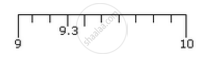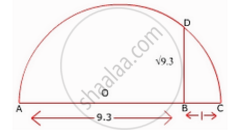# Represent √9.3 on the number line. - Mathematics

Represent sqrt9.3  on the number line.

#### SolutionMark the distance 9.3 from a fixed point A on a given line to obtain a point B such that

AB = 9.3 units. From B mark a distance of 1 unit and mark the new point as C. Find the mid-point of AC and mark that point as O. Draw a semi-circle with centre O and radius OC. Draw a line perpendicular to AC passing through B and intersecting the semi-circle at D. Then BD = sqrt9.3" ."Concept: Operations on Real Numbers
Is there an error in this question or solution?
Chapter 1: Number Systems - Exercise 1.5 [Page 24]

#### APPEARS IN

NCERT Class 9 Maths
Chapter 1 Number Systems
Exercise 1.5 | Q 4 | Page 24

Share